Previous Chapter

### The Consumer: Marginal Value, Marginal Utility, and Consumer Surplus

In Chapter 3 we used geometry, in the form of budget lines and indifference curves, to analyze the behavior of someone consuming only two goods. In this chapter we redo the analysis for a consumer buying many goods. We again use geometry, but in a different way. Each diagram shows on its horizontal axis quantity of one good, and on its vertical axis something related to that good (utility, value, marginal utility, marginal value) that varies with quantity.

We begin in the first part of the chapter by developing the concepts of marginal utility and marginal value and showing how they can be used to analyze the behavior of a consumer. The most important result of that analysis will be that the consumer's demand curve is identical to his marginal value curve. In the second part of the chapter that result will be used to derive the concept of consumer surplus--the answer to the question "How much is it worth to me to be able to buy some good at a particular price--how much better off am I than if the good did not exist?" The remainder of the chapter is a collection of loosely related sections in which I rederive the equimarginal principle, examine more carefully exactly what we have been doing in the past two chapters, and use consumer surplus to analyze the popcorn puzzle discussed in Chapter 2.

MARGINAL UTILITY AND MARGINAL VALUE

So far, we have considered the consumption of only two goods--simple to graph but hardly realistic. We shall now consider the more general case of many goods. Since we only have two-dimensional graph paper, we imagine varying the quantity of one good while spending whatever income we have left on the optimal bundle of everything else.

Table 4-1 shows bundles, each of which contains the same quantity of all goods other than oranges, plus some number of oranges. In addition to showing the utility of each bundle, it also shows the marginal utility for each additional orange--the increase in utility as a result of adding that orange to the bundle. Figure 4-1 shows the same information in the form of a graph, with number of oranges on the horizontal axis and total utility and marginal utility on the vertical axes. In comparing the table to the figure, you will note that on the table there is one value of marginal utility between 1 orange and 2, another between 2 and 3, and so forth, while on the figure marginal utility changes smoothly with quantity. The marginal utility shown on the table is really the average value of marginal utility over the corresponding range. For example, 20 is the average of marginal utility between 1 and 2 (oranges)--bundles B and C.

Table 4-1

 Bundle Oranges/Week Total Utility Marginal Utility A 0 50 B 1 80 30 C 2 100 20 D 3 115 15 E 4 125 10 F 5 133 8 G 6 139 6 H 7 144 5 I 8 146 2 J 9 147 1 K 10 147 0 L 11 147 0 M 15 147 0 N 20 147 0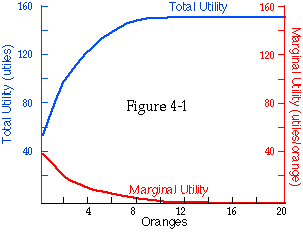Total utility and marginal utility of oranges, assuming that it costs nothing to dispose of them. Total utility is shown in black, and marginal utility is shown in color. Because surplus oranges can be freely disposed of, marginal utility is never negative, and total utility never decreases with increasing numbers of oranges.

On a table such as Table 4-1, marginal utility is the difference between the utility of 1 orange and none, between 2 and 1, and so forth. On a graph such as Figure 4-1, it is the slope of the total utility curve. Both represent the same thing--the rate at which total utility increases as you increase the quantity of oranges. Since marginal utility is the slope of total utility, it is high when total utility is rising steeply, zero when total utility is constant, and negative if total utility is falling.

Total utility is stated in utiles--hypothetical units of utility. Since marginal utility is an increase in utility divided by an increase in oranges, it is measured in utiles per orange. That is why Figure 4-1 has two different vertical axes, marked off in different units. Both marginal utility and total utility depend on quantity of oranges, so both have the same horizontal axis. By putting them on the same graph, I make it easier to see the relationship between them.

The idea of total and marginal will be used many times throughout this book and applied to at least five different things--utility, value, cost, revenue, and expenditure. In each case, the relation between total and marginal is the same--marginal is the slope of total, the rate at which total increases as quantity increases. In this chapter, we use marginal utility and marginal value in order to understand consumer choice; in later chapters, production (both by individuals and by firms) will be analyzed in a similar way using marginal cost.

#### Declining Marginal Utility

You are deciding how many oranges to consume. If the question is whether to have one orange a week or none, you would much prefer one. If the alternatives are 51 oranges a week or 50, you may still prefer the additional orange, but the gain to you from one more orange is less. The marginal utility of an orange to you depends not only on the orange and you, but also on how many oranges you are consuming. We would expect the utility to you of a bundle of oranges to increase more and more slowly with each additional orange. Total utility increasing more and more slowly means marginal utility decreasing, as you can see from Table 4-1, so marginal utility decreases as the quantity of oranges increases. This is what I earlier called the principle of declining marginal utility. There may be some point (9 oranges a week on Table 4-1 and Figure 4-1) at which you have as many oranges as you want. At that point, total utility stops increasing; additional oranges are no longer a good. Their marginal utility is zero.

As long as one of the things we can do with oranges is throw them away, we cannot be worse off having more oranges; so oranges cannot be a bad. If it were costly to dispose of oranges (imagine yourself buried in a pile of them), then at some point the marginal utility of an additional orange would become negative--you would prefer fewer to more. Figure 4-2 shows your total and marginal utility for oranges as a function of the quantity of oranges you are consuming, on the assumption that it is costly to dispose of oranges.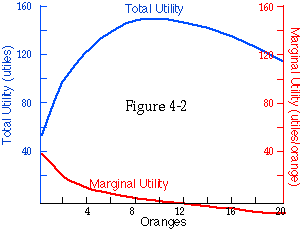Total utility and marginal utility of oranges, assuming that it is costly to dispose of them. I want to eat only 10 oranges, so additional oranges have negative marginal utility. Total utility falls as the number of oranges increases beyond 10.

#### From Marginal Utility to Marginal Value

Utility is a convenient device for thinking about choice, but it has one serious limitation--we can never observe it. We can observe whether bundle X has more utility to you than bundle Y by seeing which you choose, but that does not tell us how much more utility the bundle you prefer has. Since utiles are not physical objects that we can handle, taste, trade, and measure, we can never try the experiment of offering you a choice between an apple and 3 utiles in order to see whether the marginal utility of an apple to you is more or less than 3.

What we can observe is the relative marginal utilities of different goods. If we observe that you prefer 2 apples to 1 orange, we can conclude that the additional utility you get from the 2 apples is more than you get from the orange; hence the marginal utility per apple must be more than half the marginal utility per orange. If instead of measuring utility in utiles we measure it in units of the marginal utility of 1 apple, we can then say that the marginal utility of 1 orange is less than 2. If we observe that you are indifferent between 3 apples and 1 orange, we can say that the marginal utility of an orange is exactly 3.

What we are now dealing with is called marginal value; it is what one more unit of a good is worth to you in terms of other goods. Unlike marginal utility, it is in principle (and to some extent in practice) observable. We cannot watch you choose between apples and utiles, but we can watch you choose between apples and oranges. It is what I referred to in the previous chapter as the value of an orange (measured in apples). A more precise description would have been "the value of one more orange."

While we could discuss marginal value in terms of apples, it is easier to discuss it in terms of dollars. "The value to you of having one more orange is \$1" means that you are indifferent between having one more orange and having one more dollar. Since the reason we want money is to buy goods with it, that means that you are indifferent between having one more orange and having whatever goods you would buy if your income were \$1 higher. A graph showing total and marginal utility (Figure 4-2) and the corresponding graph showing total and marginal value (Figure 4-3) appear the same, except for the scale; the vertical axis of one has utiles where the other has dollars, and \$1 need not correspond to one utile. In drawing the figures, I have assumed that the marginal utility of income is 2 utiles/dollar (an additional \$1 is worth 2 utiles), so a marginal utility of 20 utiles per orange corresponds to a marginal value of \$10/ orange, and a total utility of 60 utiles corresponds to a total value of \$30.

This is an adequate way of looking at the relation between marginal value and marginal utility so long as we only consider situations in which the marginal utility of \$1 does not change. If it does, then measuring utility in dollar units is like measuring a building with a rubber ruler. The resulting problems will be discussed in the optional section at the end of this chapter. For the moment, we will assume that the marginal utility of \$1 can be treated as a constant. In that case, marginal value is simply marginal utility divided by the marginal utility of an additional dollar of income:

MV(oranges) = MU(oranges)/MU(income).

How Are Marginal Eggs Different From Other Eggs? You eat some number of eggs each week. Suppose that the marginal value of the fifth egg (per week) is \$0.50 (per week). This does not mean that there is a particular egg that is worth \$0.50; it means that the difference between having 5 eggs per week and having 4 is worth \$0.50/week. If we imagine that 5 eggs per week means 1 egg/day from Monday through Friday (cereal on the weekend), there is no reason why any one of those eggs should be valued more than another--but it seems likely that the extra value of 5 eggs a week instead of 4 is less than the extra value of 4 instead of 3. There is a marginal value of egg, not a marginal egg.

While this is the correct way of looking at marginal value in general, there are some particular cases in which one can talk about a marginal unit--a specific unit that produces the marginal value. Considering such cases may make it easier to understand the idea of marginal value. Once understood, it can then be applied to more general cases.

The Declining Marginal Value of Water. Suppose, for example, that we use water for a number of different uses--drinking, washing, flushing, watering plants, swimming. The value of a gallon of water used in one way does not depend on how much water we are using in another; each is independent. To each use we can assign a value per gallon. If the price of water is \$1/gallon, we use it only for those uses where it is worth at least that much; as the price falls, the number of uses expands. If water is worth \$1/gallon to us for washing but only \$0.10/gallon for swimming and \$0.01/gallon for watering the lawn, then if its price is between \$0.10 and \$1 we wash but do not swim, if it is between \$0.01 and \$0.10 we wash and swim but do not water, and if it is below \$0.01 we do all three. We can then talk of the marginal use for water--the use that is just barely worthwhile at a particular price.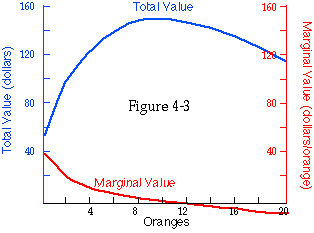Total value and marginal value of oranges. The marginal utility of income is assumed to be 2 utiles per dollar, so total value is half as many dollars as total utility is utiles. The same is true for marginal value and marginal utility.

If each additional unit of water goes for a different and independent use, there is an obvious justification for the principle of declining marginal utility. If you have only a little water, you use it for the most valuable purposes--drinking, for example. As you increase your consumption, additional water goes into less and less important uses, so the benefit to you of each additional gallon is less than that of the gallon before. In this particular case, declining marginal utility is not merely something we observe but also something implied by rationality. The difference between this and the egg case is that using water for a swimming pool does not change the value to us of using water to drink or to water the lawn, whereas eating an egg every Wednesday, in addition to the Monday, Tuesday, Thursday, and Friday eggs, may make us enjoy the other four eggs a little less.

The Declining Marginal Value of Money. Consider, instead of water, money. There are many different things you can buy with it. Imagine that all of the things come in \$1 packages. You could imagine arranging the packages in the order of how much you valued them--their utility to you. If you had \$100, you would buy the 100 most valuable packages. The more money you had, the further down the list of packages you could go and the less valuable the marginal package would be. So additional money is worth less to you the more money you have.

While this way of looking at things is useful, it is not entirely correct, since goods are not independent; the possession of one may make another more or less valuable. One can imagine situations in which increasing your income from \$3,000/year to \$3,001 was more important than increasing it from \$2,000 to \$2,001. You may find it interesting to think up some examples. I will return to the subject in a later chapter.

#### Marginal Value and Demand

Figures 4-4c and 4-4d show the same relation for a continuous good. As long as you are consuming a quantity of wine for which the marginal value of additional wine is greater than its price, you can make yourself better off by increasing your consumption. So you buy that quantity for which marginal value equals price. Since you do that for any price, your demand curve and your marginal value curve are the same.

By the principle of declining marginal utility, the marginal value curve should slope down; the more we have, the less we value additional quantities. I have just demonstrated that the demand curve is identical to the marginal value curve. It follows that demand curves slope down.Marginal value and points on the demand cruve. Panels (a) and (b) show a lumpy good. At any price, you buy a quantity for which marginal value equals the price, so the (price, quantity) points on the demand curve are the same as the (marginal value, quantity) points on the marginal value curve. Panels (c) and )d) show a continuous good. At any price, you buy a quantity for which marginal value equals the price; that is true for every price, so the demand curve is identical to the marginal value curve.

Some Problems. There is one flaw in this argument. So far, I have been assuming that the marginal utility of income--the increased utility from the goods bought with an extra dollar--is constant. But just as the marginal utility of apples depends on how many apples we have, the marginal utility of income depends on how much income we have. If our income increases, we will increase the quantities we consume (for normal goods), reducing the marginal utility of those goods. The marginal utility of a dollar is simply the utility of the additional goods we could buy with that dollar; so as income rises, the marginal utility of income falls.

A marginal value curve shows us what happens when we increase our consumption of one good while holding everything else constant. This does not quite correspond to what is shown by the demand curve of Figure 4-4d. That curve graphs quantity against price. As the price of the good falls and the quantity consumed increases, the total amount spent on that good changes--and so does the amount left to spend on other goods. Since the marginal value curve shows the value of a good measured in money, it should shift slightly as the change in that good's price changes the amount we have left to spend on other goods, and hence the marginal utility of money.

A similar difficulty in the analysis arises when the value to us of one good depends on how much we have of some other good. Bread is more valuable when we have plenty of butter, and butter less valuable when we have plenty of margarine. As price falls and quantity consumed rises in Figures 4-4b and 4-4d, the quantities of other goods consumed changes--which may affect the value of the good whose price has changed.

The problems here are the same as in the case of the Giffen good discussed earlier; a change in the price of one good affects not only the cost of that good in terms of others but also the consumer's total command over goods and services--a drop in price is equivalent to an increase in income. A full discussion of this would involve the income-compensated (Hicksian) demand curve discussed in the previous chapter.

A simpler solution, adequate for most practical purposes, is the one we used to justify the downward-sloping demand curve in the previous chapter. Since consumption is usually divided among many different goods, with only a small part of our income spent on any one, a change in the price of one good has only a very small effect on our real income and our consumption of other goods as compared to its effect on the cost of the good whose price has changed. If we ignore the small income effect, the complications of the last few paragraphs disappear. The demand curve is then exactly the same as the marginal value curve; since the latter slopes down (because of diminishing marginal utility), so does the former. The indifference curve argument gave us a downward-sloping demand curve for a consumer choosing between two goods; this argument gives one in the general case of a consumer buying many goods.

Warning. When I ask students taking an exam or quiz to explain why the demand curve is the same as the marginal value curve, most of them think they know the answer--and most of them are wrong. The problem seems to be a confusion based on an imprecise verbal argument. It sounds very simple: "Your demand is how much you demand something, which is the same as how much you value it" or, alternatively, "Your demand is how much you are willing to pay for it, which is how much you value it." But both of those explanations are wrong. Your demand curve shows not how much you demand it but how much of it you demand--a quantity, not an intensity of feeling.

Your demand curve does not show how much you are willing to give for the good. On Figure 4-4d, the point X (price = \$25/gallon, quantity = 2 gallons/week) is above your demand curve. But if you had to choose between buying 2 gallons of wine a week at a price of \$25/gallon or buying no wine at all, you would buy the wine; as we will see in a few pages, its total value is more than its cost. The demand curve shows the quantity you would choose to buy at any price, given that (at that price) you were free to buy as much or as little as you chose. It does not show the highest price you would pay for any quantity if you were choosing between that quantity and nothing.

What the height of your demand curve at any price is equal to is the amount you would be willing to pay for a little more of the good--your marginal value. That is true--but not because demand and value mean the same thing. The reason was given in the discussion of eggs and wine a few paragraphs earlier. It is also important; as you will see later in the chapter, the relation between demand and marginal value is essential in deriving consumer surplus, and as you will see later in the book, consumer surplus is an important tool in much of economics. I have emphasized the relationship between the two curves so strongly because it is easy to skip over it as obvious and continue building the structure of economics with one of its foundations missing.

#### Price, Value, Diamonds, and Water

In addition to the downward-sloping demand curve, another interesting result follows from the analysis of marginal value. As I pointed out earlier, there is no obvious relation between price (what you must give up to get something) and value (how much it is worth to you--what you are willing, if necessary, to give up to get it), a point nicely summarized in the saying that the best things in life are free. But if you are able to buy as much as you like of something at a per-unit price of P, you will choose, for the reasons discussed above, to consume that quantity such that an additional unit is worth exactly P to you. Hence in equilibrium (when you are dividing your income among different goods in the way that maximizes your welfare), the marginal value of goods is just equal to their price! If the best things in life really are free, in the sense of being things of which you can consume as much as you want without giving up anything else (true of air, not true of love), then their marginal value is zero!

This brings us back to the "diamond-water paradox." Water is far more useful than diamonds, and far cheaper. The resolution of the paradox is that the total value to us of water is much greater than the total value of diamonds (we would be worse off with diamonds and no water than with water and no diamonds), but the marginal value of water is much less than that of diamonds. Since water is available at a low cost, we use it for all its valuable uses; if we used a little more, we would be adding a not very valuable use, such as watering the lawn once more just in case we had not watered it quite enough. Diamonds, being rare, get used only for their (few) valuable uses. Relative price equals relative marginal value; diamonds are much more expensive than water.

CONSUMER SURPLUS

This brings us to another (and related) paradox. Suppose you argued that "since the value of everything is equal to its price, I am no better off buying things than not buying, so I would be just as happy on Robinson Crusoe's island with nothing for sale as I am now." You would be confusing marginal value and average value; you are no better off buying the last drop of water at exactly its value but are far better off buying (at the same price) all the preceding (and to you more valuable) drops. Note that "preceding" describes order in value, not in time.

Can we make this argument more precise? Is there some sense in which we can define how much better off you are by being able to buy as much water as you want at \$0.01/gallon or as many eggs as you want at \$0.80/egg? The answer is shown in Figure 4-5a. By buying one egg instead of none, you receive a marginal value of \$1.20 and give up \$0.80; you are better off by \$0.40. Buying a second egg provides a further increase in value of \$1.10 at a cost of another \$0.80. So buying 2 eggs instead of none makes you better off by \$0.70.

This does not mean you have \$0.70 more than if you bought no eggs--on the contrary, you have \$1.60 less. It means that buying 2 eggs instead of none makes you as much better off as would the extra goods you would buy if your income were \$0.70 higher than it is. You are indifferent between having your present income and buying 2 eggs (as well as whatever else you would buy with the income) and having \$0.70 more but being forbidden to buy any eggs.

Continuing the explanation of Figure 4-5a, we see that as long as you are consuming fewer than 5 eggs per week, each additional egg you buy makes you better off. When your consumption reaches 5 eggs per week, any further increase involves buying goods that cost more than they are worth. The total gain to you from consuming 5 eggs at a price of \$0.80 each instead of consuming no eggs at all is the sum of the little rectangles shown in the figure. The first rectangle is a gain of \$0.40/egg times 1 egg, for a total gain of \$0.40; the next is \$0.30/egg times 1 egg, and so on.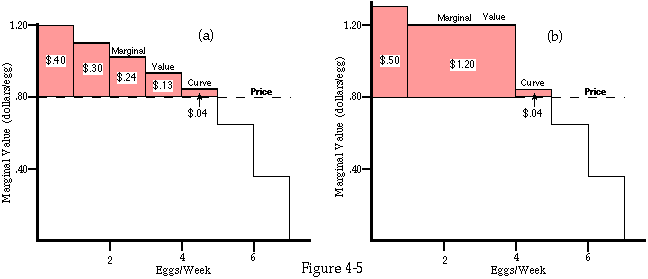Marginal value curve and consumer surplus for a lumpy good. The shaded area under the marginal value curve and above the price equals the benefit to you of buying that quantity at that price. It is called consumer surplus.

Summing the area of the rectangles may seem odd to you. Why not simply sum their heights, which represent the gain per egg at each stage? But consider Figure 4-5b, which shows a marginal value curve for which the rectangles no longer all have a width of 1 egg per week. Gaining \$0.40/egg on 3 eggs is worth 3 times as much as gaining \$0.40/egg on 1 egg.

Finally, consider Figure 4-6a, where instead of a lumpy good such as eggs we show a continuous good such as wine (or apple juice). If we add up the gain on buying wine, drop by drop, the tiny rectangles exactly fill the shaded region A. That is your net gain from being able to buy wine at \$8/gallon.

This area--representing the gain to a consumer from what he consumes--has a name. It is called consumer surplus. It equals the area under the demand curve and above the price-area A on Figure 4-6a. You will meet consumer surplus again--its derivation was one of the main purposes of this chapter. Its traditional use in economics is to evaluate the net effect on consumers of some change in the economic system, such as the introduction of a tax or a subsidy. As we will see in Chapters 10 and 16, it is also sometimes useful for helping a firm decide how to price its product.

Your consumer surplus from buying wine at some price is the value to you of being able to buy as much wine as you wish at that price--the difference between what you pay for the wine and what it is worth to you. The same analysis can be used to measure the value to you of other opportunities. Suppose, for example, that you are simply given 2 gallons per week for free, with no opportunity to either sell any of it or buy any more. The value to you of what you are getting is the value of the first drop of wine, plus the second, plus ... adding up to the whole area under your demand curve--region A plus region B on Figure 4-6a. The situation is just the same as if you bought 2 gallons per week at a price of \$8/gallon and were then given back the money. Area A is the consumer surplus on buying the wine; area B is the \$16/week you spend to get it. The total value to you of the wine is the sum of the two, which is the area under the marginal value curve; total value is simply the area under marginal value.Marginal value and consumer surplus for a continuouos good. A is the consumer surplus from beijng able to buy all the wine you want at \$8/gallon. B is what you pay for it. A+B is the total value to you of 2 gallons per week of wine. B+E+D is what you would pay if you bought 2 gallons per week at \$25/gallon.

If area A plus area B is the value to you of being given 2 gallons of wine per week, it is also the largest sum you would pay for 2 gallons per week if the alternative were having no wine at all. Figure 4-6b shows that situation. Your surplus from buying 2 gallons per week for \$25/gallon is the value to you of the wine--areas A plus B on the previous figure, equal to C + E + B on Figure 4-6b--minus what you spend for it. You are spending \$25/gallon and buying 2 gallons, so that comes to \$50/week--the colored rectangle D + E + B on Figure 4-6b. Subtracting that from the value of the wine (C + E + B) gives a surplus equal to region C minus region D. Your surplus is positive, so you buy the wine. This is the case mentioned earlier in the chapter where you would rather have a price/quantity combination that is above your demand curve than have nothing.

ODDS AND ENDS

#### Again the Equimarginal Principle

I have proved that if the marginal utility per dollar's worth of the different goods you are consuming is not the same, you must not be choosing the optimal bundle. So if you are choosing the optimal bundle, the marginal utility of a dollar's worth of any of the goods you consume must be the same. In other words, the marginal utility of each good must be proportional to its price. If butter costs \$4/pound and gasoline \$2/gallon, and a dollar's worth of butter (1/4 pound) increases your utility by the same amount as a dollar's worth of gasoline (1/2 gallon), the marginal utility of butter (per pound) must be twice the marginal utility of gasoline (per gallon)--just as the price of butter (per pound) is twice the price of gasoline (per gallon).

This is now the fourth time I have derived this result. The third was when, in the process of showing that the marginal value curve and the demand curve are the same, I demonstrated that you consume any good up to the point where its marginal value is equal to its price. While I did not point out then that marginal value equal to price implies the equimarginal principle, it is easy enough to see that it does. Simply repeat the argument for every good you consume. If marginal value is equal to price for every good, then for any two goods, the ratio of their marginal values is the same as the ratio of their prices. Since marginal value is marginal utility divided by the marginal utility of income, the ratio of the marginal values of two goods is the same as the ratio of their marginal utilities.

This may be clearer if it is stated using algebra instead of English. Consider two goods X and Y, with marginal values MVx , and MVy , marginal utilities MUx and MUy , and prices Px and Py . We have

MVx = Px;

MVy = Py;

MVx [[equivalence]] MUx/MU(income);

MVy [[equivalence]] MUy/MU(income).

Therefore,

Px/Py = MVx/MVy = MUx/MUy.

The left hand side of this equation corresponds to "the price of an apple measured in oranges" in Chapter 3 (minus the slope of the budget line; apples are X, oranges Y); the right hand side is the marginal rate of substitution (minus the slope of the indifference curve).

This is the final derivation of the principle in this chapter, but you will find it turning up again in economics (and elsewhere). The form in which we have derived it this time makes more obvious the reason for calling it the equimarginal principle. A convenient, if sloppy, misstatement of it is "Everything is equal on the margin."

It is important, in this and other applications of the equimarginal principle, to realize that it is a statement not about the initial situation (preferences, market prices, roads, checkout counters, or whatever) but about the result of rational decision. You may (as I do) vastly prefer Kroger chocolate chip cookies (the kind they used to bake in the store and sell in the deli section) to apples; if so, you may buy many more cookies than apples. What the equimarginal principle tells you is that you will buy just enough more cookies to reduce the marginal utility per dollar of cookies to that of apples.

It may occur to some of you that there is a problem with the most recent argument by which I "proved" the equimarginal principle. I originally defined the marginal utility of something of which I have n units as the utility of n + 1 units minus the utility of n units; since marginal value is derived from marginal utility, it would be defined similarly. Applying this to my example of 9 apples and 4 cookies, the marginal value of an apple involves the difference between 9 and 10 and the marginal value of a cookie involves the difference between 4 and 5. But the change that I considered involved increasing the consumption of apples from 9 not to 10 but to 11, and decreasing the consumption of cookies from 4 to 3. Unless the marginal value of the eleventh apple is the same as that of the tenth (which it should not be, by our assumption of declining marginal utility) and the marginal value of the fourth cookie the same as that of the fifth (ditto), the argument as I gave it is wrong!

The answer to this objection is that although I have described the marginal utility of an apple or an orange as the difference between the utility of 10 and the utility of 9, that is only an approximation. Strictly speaking, we should think of all goods as consumed in continuously varying quantities (if this suggests applesauce and cookie crumbs, wait for the discussion of time in the next section). We should define the marginal utility as the increased utility from consuming a tiny bit more, divided by the amount of that tiny bit (and similarly for marginal value). Marginal value is then the slope of the graph of total value; in Figure 4-7 it is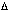V/Q. If, when we are consuming 100 gallons of water per week, an additional drop (a millionth of a gallon) is worth one hundred-thousandth of a cent, then the marginal value of water is .00001 cents/.000001 gallons, which comes out to \$0.10/gallon. The argument of the previous section can then be restated in terms of an increase in consumption of .002 apples and a decrease in consumption of .001 cookies. Since we do not expect the marginal value of cookies to change very much between 4 cookies and 3.999 cookies, the argument goes through.

The precise definitions of marginal utility (see the optional section of Chapter 3) and marginal value require calculus--the marginal value of apples is the derivative of total value with respect to quantity. Since I am not assuming that all of my readers know calculus, I use the sort of imprecise language given above. Precisely the same calculus concept (a derivative) is implicit in such familiar ideas as speed and acceleration. You might carelessly say that, having driven 50 miles in an hour, your speed was 50 miles per hour--but you know that speed is actually an instantaneous concept and that 50 miles per hour is only an average (part of the time you were standing still at a stop light, part of it going at 50, part of it at 65). A precise definition of speed must be given in terms of small changes in distance divided by the small amounts of time during which they occur, just as a precise definition of marginal value is given in terms of small changes in value divided by the small changes in quantity that cause them.

#### Economics and Time

In talking or writing about economics, it is often convenient to describe consumption in terms of quantities--numbers of apples, gallons of water, and so forth. But 100 apples consumed in a day are not of the same value to me as 100 apples consumed in a year. The easiest way to deal with this problem is to think of consumption in terms of rates instead of quantities--6 apples per week, 7 eggs per week, and so on. Income is not a number of dollars but rather a number of dollars per week. Value is also a flow--6 apples per week are worth, not \$3, but \$3/week.

If we think of all quantities as flows and limit ourselves to analyzing situations in which income, prices, and preferences remain the same for long periods, we avoid most of the complications that time adds to economics. Many of these complications are important to understanding the nonstatic world we live in. But in solving a hard problem, it is often wise to solve the easier parts first; so in this section of the book, the problems associated with change are mostly ignored. Once we have a clearly worked-out picture of static economics, we can use it to understand more complicated situations--and will, starting in Chapter 12. Until then, we are doing economics in a perfectly static and predictable world, in which tomorrow is always like today and next year is always like this year. That is why, in drawing indifference curve diagrams, we never considered the possibility that the consumer would spend only part of his income in order to save the rest for a rainy day; either it is raining today or there are no rainy days.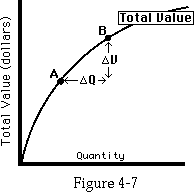Total value and its slope.V/Q is the average slope of total value between A and B. AsV andQ become very small, A and B move together, andV/Q approaches the slope of total value at a point-which is marginal value.

Problems associated with time and change are not the only complications ignored at this point; you might find it interesting to make a list as we go along, and see how many get dealt with by the end of the book.

One advantage to thinking of consumption in eggs per year instead of just eggs is that it lets us vary consumption continuously. There are severe practical difficulties with changing the number of eggs you consume by 1/10 of an egg at a time--what do you do with the rest of it? But it is easy enough to increase the rate at which you consume eggs by 1/10 of an egg per week--eat, on average, 5 more eggs per year. Thus lumpy goods become continuous--and the consumption of continuous goods is, for mathematical reasons, easier to analyze than the consumption of lumpy goods. We can then define marginal utility and marginal value in terms of very small amounts of apples and cookies without first converting the apples into applesauce and the cookies into a pile of crumbs.

There is a second problem associated with time that we should also note. In describing the process of choice, I talk about "doing this, then doing that, then . . . " For example, I talk about increasing consumption from 4 apples to 5, then from 5 to 6, then from . . . and so on. It sounds as though the process happens over time, but that is deceptive. We are really describing not a process of consumption going on out in the real world but rather something happening inside your head--the process of solving the problem of how much of each good to consume. A more precise description would be "First you imagine that you choose to consume no apples and consider the resulting bundle of goods. Then you imagine that you consume 1 apple instead of none and compare that bundle with the previous one. Then 2 instead of 1. Then . . . . Finally, after you have figured out what level of consumption maximizes your utility, we turn a switch, the game of life starts, and you put your solution into practice."

If you find it difficult to distinguish time in the sense of an imaginary series of calculations by which you decide what to do from the time in which you actually do it, you may instead imagine, as suggested before, that we are considering a situation (income, preferences, prices) that will be stable for a long time. We start by spending a few days experimenting with different consumption bundles to see which we prefer. The loss from consuming wrong bundles during the experiment can be ignored, since it is such a short period compared to the long time during which the solution is put into practice.

#### Money, Value, and Prices

Although prices and values are often given in terms of money, money has nothing essential to do with the analysis. In demonstrating the equimarginal principle, for example, I converted cookies into money (bought one less cookie, leaving me with an extra dollar to spend on something else) and then converted the money into apples (bought 2 apples for \$1). The argument would have been exactly the same if there were no such thing as money and a cookie simply exchanged for 2 apples.

We are used to stating prices in money, but prices can be stated in anything of value. We could define all our prices as apple prices. The apple price of a cookie, in my example, is 2 apples--that is what you must give up to get a cookie. The apple price of an apple is 1 (apple). Once you have the price of everything in terms of apples, you also have the price of everything in terms of any other good. If a peach exchanges for 4 apples, and 4 apples exchange for 8 cookies, then the cookie price of a peach is 8.

This argument depends on an assumption that has so far been implicit in our analysis--that we can ignore all costs of buying and selling other than the price paid. This assumption, sometimes called zero transaction costs, is a reasonable approximation for much of our economic activity and one that will be retained through most of the book. Exceptions are discussed in parts of Chapters 6 and 18. It is not clear that the assumption is reasonable here. Imagine, for example, that you have 20 automobiles and want a house. The cookie price of an automobile is 40,000; the cookie price of a house is 800,000. It seems, from the discussion of the previous paragraph, that all you have to do to get your house is trade automobiles for cookies and then cookies for the house.

But where will you put 800,000 cookies while you wait for the seller of the house to come collect them? How long will it take you to count them out to him? What condition will the cookies be in by the time you finish? Clearly, in the real world, there are some problems with such indirect transactions.

This brings us to the second reason why relative prices--prices of goods in terms of other goods--must fit the pattern I have described. Trading huge quantities of apples, cookies, peaches, or whatever may be very costly for you and me. It is far less costly for those in the business of such trading--people who routinely buy and sell carload lots of apples, wheat, pork bellies, and many other outlandish things and who make their exchanges not by physically moving the goods around but merely by changing the pieces of paper saying who owns what, while the goods sit still. For such professional traders, the assumption of zero transaction costs is close to being correct. And such traders, in the process of making their living, force relative prices into the same pattern as would consumers with zero transaction costs--even if they never consume any of the goods themselves.

I have now shown that the price of peaches in terms of cookies is determined once we know the price of both goods in apples--precisely, if transaction costs are zero; approximately, if they are not. By similar arguments, we could get the exchange ratio between any two goods (how many of one must you give for one of the other) starting with the price of both of them in apples, or in potatoes, or in anything else. The equimarginal principle then appears as "the ratio of marginal utilities of two goods is the same as their exchange ratio." If 2 apples exchange for 1 cookie, then in equilibrium a cookie must have twice the marginal utility of an apple.

I used money in talking about values as well as in talking about prices. Here too, the money is merely a convenient expository device. The statement that the marginal value of something is \$0.80 means that you are indifferent between one more unit of it and whatever else you would buy if you had an additional \$0.80. Just as in the case of prices, the money serves as a conceptual intermediate--we are really comparing one consumption good with another. The arguments of this chapter could be made in "potato values" just as easily as in "dollar values." Indeed potato values are more fundamental than dollar values, as you can easily check by having a hamburger and a plate of french-fried dollars for lunch.

It is often asserted that economics is about money or that what is wrong with economics is that it only takes money into account. That is almost the opposite of the truth. While money does play an important role in a few areas of economics such as the analysis of business cycles, price theory could be derived and explained in a pure barter economy without ever mentioning money.

A similar error is the idea that economists assume everyone wishes to maximize his wealth or his income. Such an assumption would be absurd. If you wished to maximize your wealth, you would never spend any money except for things (such as food) that you required in order to earn more money. If you wished to maximize your income, you would take no leisure (except that needed for your health) and always choose the highest paying job, independent of how pleasant it was. What we almost always do assume is that everyone prefers more wealth to less and more income to less, everything else held constant. To say that you would like a raise is not the same thing as to say that you would like it whatever its cost in additional work.

#### Conclusion: Consumption, Languages, and All That

In my analysis of consumption (Chapters 3 and 4), I have tried to do two things. The first is to show how rational behavior may be analyzed in a number of different ways, each presenting the same logical structure in a different language. The second is to use the analysis to derive three interrelated results.

The simplest of the three, derived once with indifference curves and once with marginal value, is that demand curves slope down--the lower the price of something, the more you buy. In both cases, the argument depends on declining marginal utility. In both cases, there is a possible exception, based on the ambiguity between a fall in price and a rise in income; in both cases, the ambiguity vanishes if we insist on a pure price change--a change in one price balanced by either a change in the other direction of all other prices or a corresponding change in income. It also vanishes if we assume that any one good makes up a small enough part of our consumption that we may safely ignore the effect on our real income of a change in its price.

A second result is that the value to a consumer of being able to buy a good at a price, which we call consumer surplus, equals the area under the demand curve and above the price. At this point, that may seem like one of those odd facts that professors insist, for their own inscrutable reasons, on having students memorize. I suggest that instead of memorizing it, you go over the derivation of that result (eggs and wine) until it makes sense to you. At that point, you will no longer need to memorize it, since you will be able to reproduce the result for yourself. It is worth understanding, and not just for passing economics courses. As we will see in later chapters, consumer surplus is the essential key to understanding arguments about policy ("should we have tariffs?") as well as to figuring out how to maximize profits at Disneyland.

The third result from these chapters is the equimarginal principle, which tells us that, as a result of our own rational behavior, the ratio of the marginal utilities of goods is the same as the ratio of their prices. In addition to helping us understand consumption, the equimarginal principle in this guise is one example of a pattern that helps us understand how the high salaries of physicians are connected to the cost of medical school and the labors of interning, why we do not get ahead by switching lanes on the freeway, and how not to make money on the stock market.

#### POPCORN-AN APPLICATION

In Chapter 2, I asked why popcorn is sold at a higher price in movie theaters than elsewhere. While we will not be ready to discuss possible right answers until Chapter 10, we can at this point use the idea of consumer surplus to show that the obvious answer is wrong. The obvious answer is that once the customers are inside the theater, the owner has a monopoly; by charging them a high price, he maximizes his profit. What I will show is that far from maximizing profits, selling popcorn at a high price results in lower profits than selling popcorn at cost!

To do this, I require the usual economic assumption that people are rational, plus an important simplifying assumption--that all consumers are identical. While the latter assumption is unrealistic, it should not affect the monopoly argument; if the theater owner charges high prices because he has a monopoly, he should continue to do so even if the customers are all the same. Here and elsewhere, the assumption of identical consumers (and identical producers) very much simplifies our analysis. It is frequently a good way of getting a first approximation solution to an economic problem.

The theater owner is selling his customers a package consisting of the opportunity to watch a film, plus associated goods such as comfortable seats, clean rest rooms, and the opportunity to buy popcorn. He charges his customers the highest price at which he can sell the package. Since the customers are identical, there is one price that everyone will pay and a slightly higher price that no one will pay.

In order to decide what to put into the package, the owner must consider how changes will affect its value to the customers and hence the maximum he can charge the customers for a ticket. Suppose, to take a trivial case, he decides to improve the package by giving every customer a quarter as he comes in the door. Obviously this will increase the amount the customers are willing to pay for a ticket by exactly \$0.25. The owner is worse off by the time and trouble spent handing out the coins.

Suppose the theater owner decides that since he has a monopoly on providing seats in the theater, he might as well charge \$1 for each seat in addition to the admission price. Since everyone wants a seat, the consumer is paying (say) \$4 for an admission ticket and another \$1 for a seat. That is the same as paying \$5 for admission. If the customer is not willing to pay \$5 for the movie, he will be no more willing when the payment is divided into two pieces; if he is willing to pay \$5, the theater owner should have been charging \$5 in the first place.

Now suppose the theater owner is trying to decide whether to sell popcorn in the theater at \$1/carton or not sell it at all. One advantage to selling popcorn is that he gets money for the popcorn; another is that customers prefer a theater that sells popcorn to one that does not and are therefore willing to pay more for admission. How much more?

Figure 4-8 shows a customer's demand curve for popcorn. At \$1/carton, he buys 1 carton. The shaded area is his consumer surplus--\$0.25. That means (by the definition of consumer surplus) that the customer is indifferent between being able to buy popcorn at \$1/carton and being unable to buy any popcorn but being given \$0.25; the opportunity to buy popcorn at \$1/carton is worth \$0.25 to him. Making the popcorn available at that price is equivalent to handing each customer a quarter as he walks in the door; it makes the package offered by the theater (movie plus amenities--including popcorn) \$0.25 more valuable to him, so the theater owner can raise the admission price by \$0.25 without driving off the customers. The owner should start selling popcorn, provided that the cost of doing so is less than \$1.25/customer. That is what he gets from selling the popcorn--a dollar paid for the popcorn plus \$0.25 more paid for admission because the opportunity to buy popcorn is now part of the package.One theater customer's demand curve for popcorn. The shaded triangle is the consumer surpluse frombuying popcorn at \$1/carton. The colored region (ABEDC) is the increase in his consumer surplus if price falls from \$1/carton to \$0.50/carton.

Is \$1/carton the best price? Assume that, as shown on Figure 4-8, the marginal cost to the owner of producing popcorn (the additional cost for each additional carton produced) is \$0.50/carton. He can produce as many cartons as he likes, at a cost of \$0.50 (for popcorn, butter, wages, and so forth) for each additional carton. Suppose he lowers the price of popcorn from \$1 to \$0.50. He is now selling each customer 2 cartons instead of 1, so his revenue is still \$1/customer. His costs have risen by \$0.50/customer, since he has to produce 2 cartons instead of 1. Consumer surplus, however, has risen by the colored area on Figure 4-8, which is \$0.75; he can raise the admission price by that amount without losing customers. His revenue from selling popcorn is unchanged, his costs have risen by \$0.50/ customer, and his revenue from admissions has risen by \$0.75/customer; so his profits have gone up by \$0.25/customer.

The argument is a general one; it does not depend on the particular numbers I have used. As long as the price of popcorn is above its marginal cost of production, profit can be raised by lowering the price of popcorn to marginal cost (MC on Figure 4-8) and raising the price of admission by the resulting increase in consumer surplus. The reduction in price reduces the owner's revenue on the popcorn that he was selling already by its quantity times the reduction--rectangle ABDC. The cost of producing the additional popcorn demanded because of the lower price is just covered by what the consumers pay for it, since the price of a carton of popcorn is equal to the cost of producing it; on Figure 4-8, both the additional cost and the additional revenue from selling popcorn are rectangle DEHG. Consumer surplus goes up by the colored area in the figure--rectangle ABDC plus triangle BDE. Since the owner can raise his admission price by the increase in consumer surplus, his revenue goes up by (ABDC + BDE) (increased admission) + (DEHG - ABDC) (change in revenue from selling popcorn). His cost goes up by DEHG, so his profit goes up by the area of triangle BDE.

The same argument can be put in words, without reference to the diagram: "So far as the popcorn already being sold is concerned, the price reduction is simply a transfer from the theater owner to the customer, so revenue from selling popcorn goes down by the same amount that consumer surplus goes up (ABDC). So far as the additional popcorn sold at the lower price is concerned, the customer pays the owner its production cost (DEHG) and is left with its consumer surplus (BDE). So if we lower the price of popcorn to its marginal cost, consumer surplus goes up by more than revenue from popcorn goes down. The theater owner can transfer the consumer surplus to his own pocket by raising the admission price to the theater; by doing so (and reducing popcorn to cost), he increases his profit by the consumer surplus on the additional popcorn (BDE)."

This shows that any price for popcorn above production cost lowers the profits of the theater owner, when the effect of the price of popcorn on what customers are willing to pay to come to the theater is taken into account.

We are now left with a puzzle. We have used economics to prove that a theater owner maximizes his profits by selling popcorn at cost. Economics also tells us that theater owners should want to maximize their profits and know how to do so. That implies that they will sell popcorn at cost. Yet they apparently do not. Something is wrong somewhere; there must be a mistake either in the logic of the argument, in its assumptions, or in our observation of what theaters actually do. We will return to that puzzle, and two possible solutions, in Chapter 10.

#### CONSUMER SURPLUS AND MEASURING WITH A (SLIGHTLY) RUBBER RULER

In using the equality between the marginal value curve and the demand curve to derive a downward-sloping demand curve earlier in this chapter, I discussed some of the problems of measuring value in goods instead of in utility. We are now in a position to see how the same problem affects the concept of consumer surplus.

Suppose a new good becomes available at price P. Consumer surplus, the area under the demand curve for the new good and above a horizontal line at P, is supposed to be the net benefit to me in dollars of being able to buy the new good--the increase in my utility divided by my marginal utility for a dollar. But as I increase my expenditure on the new good, I must be decreasing my total expenditure on all old goods. The less I spend on something, the less I consume of it; the less I consume, the greater its marginal utility. So after I have adjusted my consumption pattern to include the new good, the marginal utility of all other goods has risen. Since the marginal utility of a dollar is simply the utility of what I can buy with it, the marginal utility of a dollar has also increased. But the original discussion of marginal utility, marginal value, and consumer surplus treated the marginal utility of a dollar (usually called the marginal utility of income) as a constant.

The reason this is a good approximation for most purposes is shown in Figure 4-9. I assume that I am initially consuming 25 different goods, A-Y, and a twenty-sixth good, Z, becomes available at a price Pz. The graphs show my marginal utility for goods A, B, and Z. In the initial situation (shown by the dashed lines), I am dividing all of my income among goods A-Y in such a way that the marginal utility of an additional dollar's worth of each good is the same. The price of good A is assumed to be \$1/unit (the units could be pounds, gallons, or whatever, depending on what sort of good it is); of B, \$2/unit.

After good Z becomes available, I rearrange my expenditure so that I again have the same marginal utility per dollar on each unit. Since some of my income is now going to Z, I must be spending less on each other good, as shown by the solid lines in the figure. If I simply transferred all of the expenditure away from one good, its marginal utility per dollar would rise, the marginal utility per dollar of the other goods would stay the same, and I would no longer be satisfying the equimarginal principle and hence no longer maximizing my utility. So instead, I reduce my expenditure a little on each good, raising the marginal utility of each by the same amount. The result is that I am now consuming Qa -Qa , of good A, Qb -Qb of good B, and so forth; by the equimarginal principle we have

MU(Qa-Qa )/Pa = MU(Qb -Qb)/Pb = . . . = MU(Qz)/Pz . (Equation 1)

Since total expenditure is unchanged, the reduction in expenditure on goods A-Y must equal the new expenditure on good Z, soQaPa +QbPb + . . . = QzPz. (Equation 2)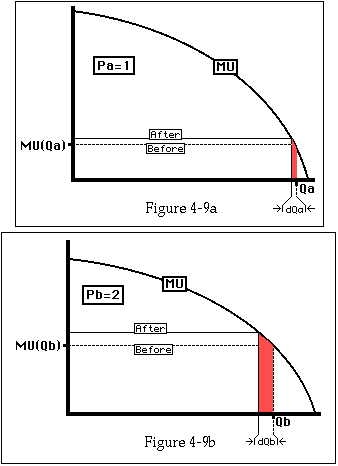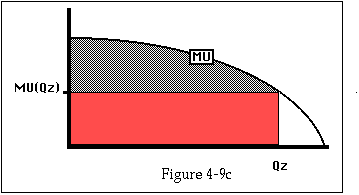Marginal utility curves for three goods, showing the situation before and after the third good becomes available. When good Z becomes available, the consumer buys less of goods A-Y and spends the money on Z instead. Colored regions show utility losses on goods A and B which (with similar losses on C-Y, not shown) add up to the colored region representing expenditure on good Z.

Since I am consuming 25 other goods, the decrease in consumption of each of them when I start consuming the new good as well is very small, as shown on the figures. So the marginal utility of a dollar's worth of the good is almost the same after the change as before.

To put the derivation of consumer surplus in terms of utility rather than in dollars (and so make it more precise), consider the narrow colored areas in Figures 4-9a and 4-9b. They represent the utility loss as a result of the decreased consumption of goods A and B. They are almost equal to the narrow rectangles whose height is MU(Q -Q)and whose width isQ, where "Q" is Qa in Figure 4-9a and Qb in Figure 4-9b. If you sum the areas of those rectangles (for all of goods A-Y), you get

Total area = MU(Qa -Qa)Qa + MU(Qb -Qb)Qb + . . . .

Substituting in from Equation 1 we have

= (MU(Qz)/Pz) x { PaQa+ PbQb + . . . ,

which by Equation 2

= (MU(Qz)/Pz)(PzQz) = MU(Qz)Qz= colored area on Figure 4-9c.

Since the total utility I get from consuming Qz of Z is the area under the MU curve (the shaded area plus the colored area) my net gain is the shaded area--my consumer surplus measured in utiles.

The one approximation in all of this was ignoring the part of the narrow rectangles on Figures 4-9a and 4-9b that was shaded but not colored. That difference becomes smaller, relative to the colored part, the larger the number of different goods being consumed; as the number of goods goes to infinity, the ratio of shaded to colored goes to zero. So consumer surplus as we measure it (the area under an ordinary demand curve and above price) and consumer surplus as we define it (the value to the consumer of being able to buy the good) are equal for a consumer who divides his expenditure among an infinite number of goods, and are nearly equal for a real consumer, who divides his expenditure among a large but finite number of goods.

A mathematical argument is not really satisfactory unless it can be translated into English. This particular one translates into a short dialogue:

Query: "When a new good becomes available, you get consumer surplus by spending money on that good. But do you not lose the consumer surplus on the other goods you are now not buying with that money?"

Response: "If you are consuming many goods, you get the money to buy the new good by giving up a marginal unit of each of the others: the last orange that was barely worth buying, the trip you weren't sure you wanted to take. The marginal unit is worth just what you pay for it--that is why it is marginal--so it generates no surplus."

PROBLEMS

1. Figure 4-10a shows a number of total utility curves and Figure 4-10b shows marginal utility curves.

a. Which total utility curves correspond to goods? (There may be more than one.)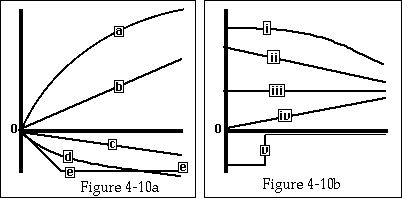Total and marginal utility curves. For Problem 1.

b. Which marginal utility curve corresponds to total utility curve b? to total utility curve e?

c. Which total utility curves and which marginal utility curves are consistent with declining marginal utility?

2. Figure 4-11a shows some total utility curves; draw the corresponding marginal utility curves.

3. Figure 4-11b shows some marginal utility curves; draw the corresponding total utility curves.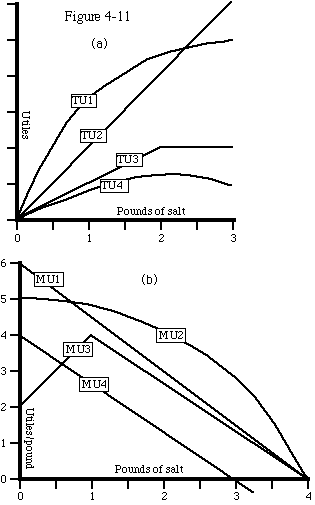Total and marginal utility curves. For Problems 2 and 3.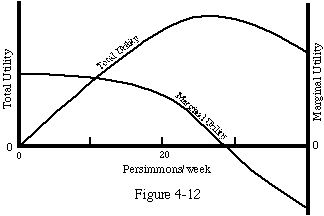Total and marginal utility curves for persimmons. For problem 4.

4. Figure 4-12 shows your marginal and total utility curves for persimmons. Are persimmons a good? A bad? Both? Explain.

5. Figure 4-13a shows your demand curve for Diet Coke.

a. Approximately how much better off are you being able to buy all the Diet Coke you want at \$5/gallon than not being able to buy any?

b. How much better off are you being able to buy all the Diet Coke you want at \$3/ gallon than at \$5/gallon?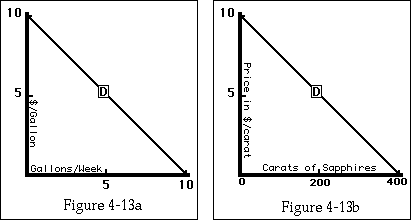Your demand curves for diet coke and sapphires. For Problems 5 and 7.

6. Estimate, to within a factor of 10, what percentage of all water used in the United States is used to drink. Give your sources. Is the common conception of a "water shortage" as a situation where people are going thirsty an accurate one? What does this tell us about the difference between the marginal value of water at a quantity of a few gallons a week and the marginal value of water at the quantity we actually consume? (The numerical part of this cannot be answered from anything in the book; it is intended to give you practice in the useful art of back-of-the-envelope calculations--very rough estimates of real-world magnitudes--while at the same time connecting the abstract examples of the chapter to something real.)

7. Figure 4-13b shows your demand curve for sapphires. For religious reasons, sapphires can neither be bought nor sold. You accidentally discover 100 carats of sapphires. How much better off are you?

8. Figure 4-14a shows your demand curve for red tape. There is no market for red tape, but the government, which is trying to reduce its inventory, orders you to buy 50 pounds of it at \$0.20/pound. How much better or worse off are you as a result?

9. You want colored marshmallows (purple, green, and gold) to put into the hot drinks at your Mardi Gras party; Figure 4-14b shows your demand curve. Colored marshmallows cost \$1/bag.

a: How many do you buy?

After you have finished buying and paying for them, there is an announcement over the store's public address system; a special Mardi Gras sale has just started, and colored marshmallows are now only \$0.50/bag.

b: Do you buy more? If so, how many?

c. What is your total consumer surplus from buying marshmallows--including those you bought initially and any others you bought during the sale?

10. In the example worked out in the text, how would profit be changed by a further reduction in the price of popcorn to \$0.25/carton?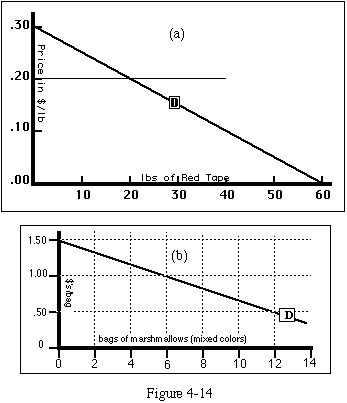Your demand curves for red tape and marshmallows. For Problems 8 and 9.

Next Chapter